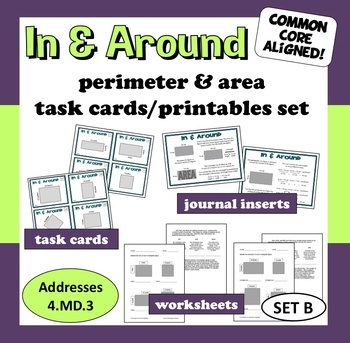# In and Around - area and perimeter task cards + printables (set B)Subject
Grade Levels
Resource Type
Product Rating
File Type

PDF (Acrobat) Document File

Be sure that you have an application to open this file type before downloading and/or purchasing.

1 MB|22 pages
Share
Product Description
Build your students’ understanding of perimeter and area with this set of task cards and printables. The 32 task cards in this set can perform double duty – providing practice with finding both perimeter and area of rectangular figures. Extend your students’ practice (or assess their mastery of perimeter and area) with the two included worksheets. With these resources, your students will grow stronger in their understanding of these key measurement concepts.

Common Core State Standards for Mathematics addressed:
Measurement and Data (4.MD)
• Apply the area and perimeter formulas for rectangles in real world and mathematical problems. (4.MD.3)

These resources are equally useful for fifth grade teachers looking to review area or third grade teachers in need of enrichment activities!

Included:
• 32 task cards
• 2 journal-sized graphic reference sheets
• 2 different answer sheets – one for perimeter, one for area
• keys for both answer sheets
• 2 two-sided worksheets – one for perimeter, one for area
• scoring guides for both worksheets

The printables consist of two journal-sized reference sheets and two different two-page worksheets that can be used to evaluate student understanding of area and perimeter. There is one reference sheet that relates to perimeter and one that relates to area. You can give these to the students before they work on the cards and allow the students to use the sheet as a reference while they complete the cards.

Each of the 32 cards presents the students with a rectangular figure. Both rectangles and squares are featured on a number of the cards as I find that even when my students consistently apply the formula for area correctly when given a rectangle, they often find the perimeter when presented with a square! The cards scale up in difficulty, moving from rectangles and squares with single-digit dimensions to ones with dimensions that are two-digit numbers. One of the answer sheets focuses on perimeter, and directs students to find and record the perimeter of each figure. The other answer sheet directs students to find and record the area of the figures. You can use them on successive or non-consecutive days, depending on the sequence of instruction you decide to use in regards to area and perimeter.

Once students have completed the task cards, you can give them the worksheet related to the skill they practiced. One worksheet assesses student understanding of perimeter and the other of area. The worksheets present students with rectangular figures (on a grid) and ask them to identify the perimeter or area. Students will then apply their knowledge of perimeter or area in real-life situations. Each worksheet has a scoring guide that spells out how many points each part of the worksheet is worth, with each worksheet having a total of 18 points.

For more practice with area & perimeter of rectangular figures, please check out the other related resources I have available –
In and Around - area and perimeter task cards + printables (set A)

I hope your students enjoy these resources and are able to build their proficiency with perimeter and area. – Dennis McDonald
Total Pages
22 pages
Answer Key
Included
Teaching Duration
N/A
Report this ResourceSign Up